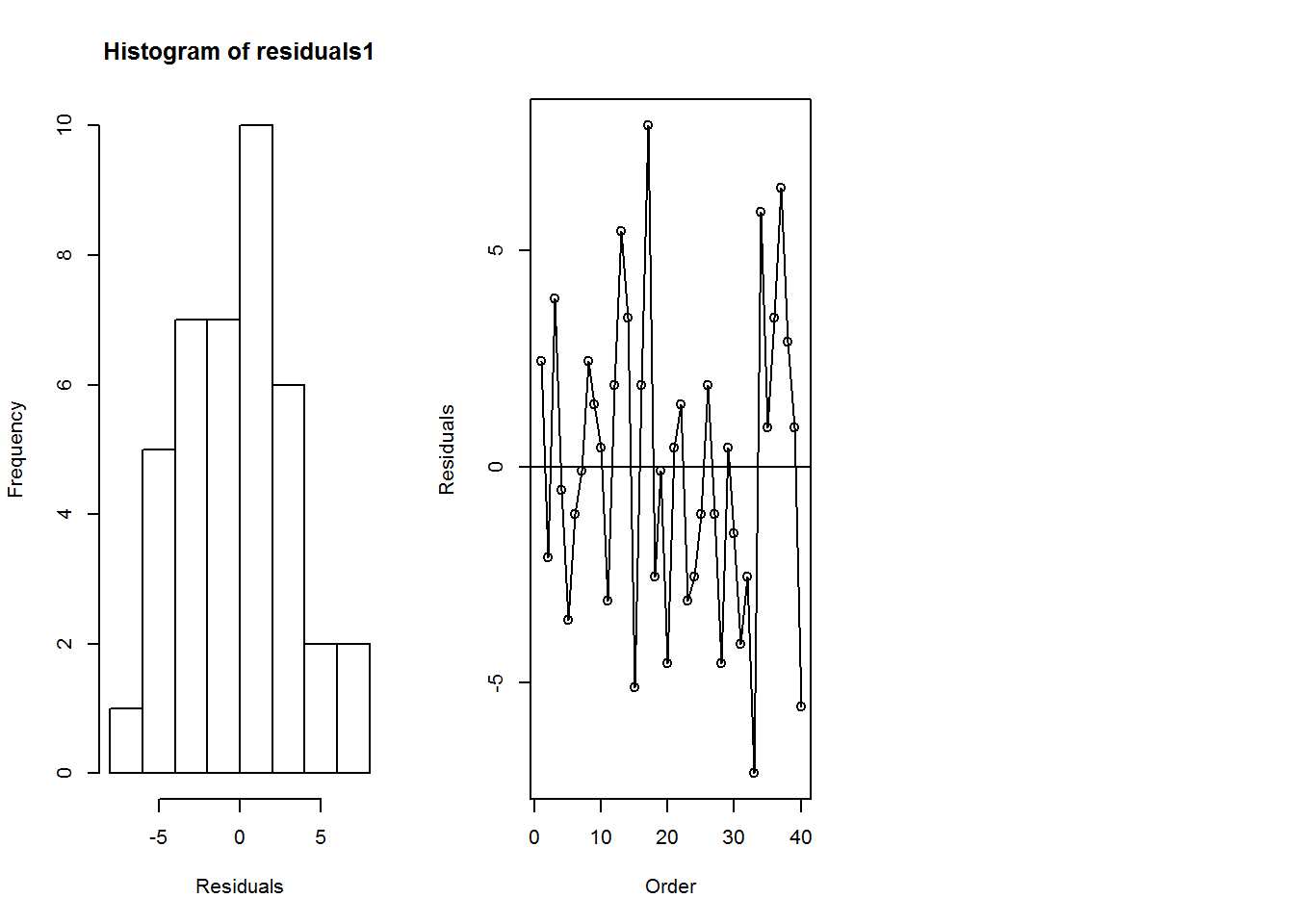require(mosaic)
## Loading required package: mosaic
## Warning: package 'mosaic' was built under R version 3.2.5
## Loading required package: dplyr
## Warning: package 'dplyr' was built under R version 3.2.5
##
## Attaching package: 'dplyr'
## The following objects are masked from 'package:stats':
##
##     filter, lag
## The following objects are masked from 'package:base':
##
##     intersect, setdiff, setequal, union
## Loading required package: lattice
## Loading required package: ggplot2
## Warning: package 'ggplot2' was built under R version 3.2.5
## Loading required package: mosaicData
## Warning: package 'mosaicData' was built under R version 3.2.5
## Loading required package: Matrix
##
## The original behavior of these functions should not be affected by this.
##
## Attaching package: 'mosaic'
## The following object is masked from 'package:Matrix':
##
##     mean
## The following objects are masked from 'package:dplyr':
##
##     count, do, tally
## The following objects are masked from 'package:stats':
##
##     binom.test, cor, cov, D, fivenum, IQR, median, prop.test,
##     quantile, sd, t.test, var
## The following objects are masked from 'package:base':
##
##     max, mean, min, prod, range, sample, sum
library(readr)
## Warning: package 'readr' was built under R version 3.2.5
#Read data set Games1 into your R session and name it "games1":

games1 <- read_csv("P:/SUNY-Brockport/SUNY Brockport_1/teaching/Staitstical Methods/Spring 2017/Chapter2/C2Games1.csv")
## Parsed with column specification:
## cols(
##   studentID = col_integer(),
##   Type = col_character(),
##   Time = col_integer()
## )
View(games1)

#Create a boxplot to compare the times for the color and standard grames:
boxplot(Time~Type,data=games1,ylab="Seconds")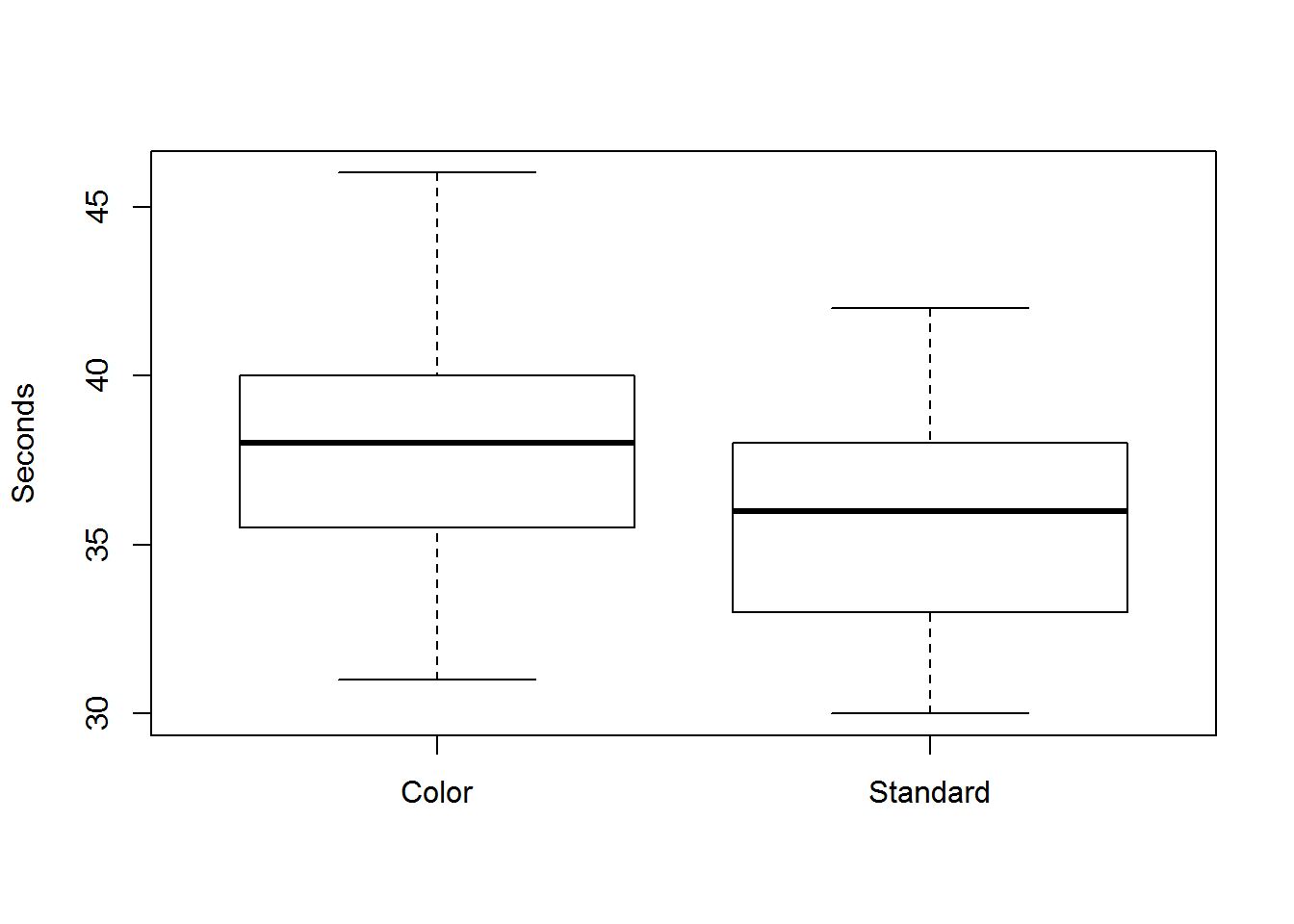#Create two vectors of Time observations for the Standard and Color groups:
Standard <- games1[games1$Type=="Standard",]$Time
Color <- games1[games1$Type=="Color",]$Time

#Mean and standard deviations for the time to win the game for standard game:
mean(Standard)
##  35.55
sd(Standard)
##  3.394655
#Mean and standard deviations for the time to win the game for color game:
mean(Color)
##  38.1
sd(Color)
##  3.654845
#Sample size, 12th observation, and 12th residual for the Standard group:
length(Standard)
##  20
Standard
##  37
Standard-mean(Standard)
##  1.45
#Sample size, 12th observation, and 12th residual for the Color group:
length(Color)
##  20
Color
##  37
Color-mean(Color)
##  -1.1
#Create a vector of residuals for all observations:
residuals <- c(Standard-mean(Standard),Color-mean(Color))

#Create a histogram of the residuals:
hist(residuals)

#Compute the ratio of the maximum of the sample standard deviations for the Standard and Color groups to
#the minimum of sample standard deviations for the Standard and Color groups:
max(c(sd(Standard),sd(Color)))/min(c(sd(Standard),sd(Color)))
##  1.076647
###############
########t-test
#Perform a two-sample t-test to compare average winning time between the Standard and Color groups
#(assuming equal variances):
t.test(Time~Type,data=games1,var.equal = TRUE)
##
##  Two Sample t-test
##
## data:  Time by Type
## t = 2.2862, df = 38, p-value = 0.02791
## alternative hypothesis: true difference in means is not equal to 0
## 95 percent confidence interval:
##  0.2920254 4.8079746
## sample estimates:
##    mean in group Color mean in group Standard
##                  38.10                  35.55
# In this chapter we are assuming equal variances. Using “var.equal = FALSE” will give the more common t-test with unequal variances.
###########################
#Regression##################

#Create a dummy variable where a value of 1 corresponds to the color distracter game:
D1 <- (games1$Type=="Color")*1 # this creates a new column 1 for Color and 0 for Standard #Regress Time on the dummy variable D1 and name the regression object games.reg1: games.reg1 <- lm(Time~D1,data=games1) #Obtain the vector of residuals: residuals <- games.reg1$res
#Estimated coefficients of the model of Time regressed on D1:
games.reg1$coef ## (Intercept) D1 ## 35.55 2.55  beta1<-games.reg1$coef

#The partial output from the following code contains the t-statistic and p-value for
#the hypothesis test that β1 0 versus the alternative β1 ≠0:
summary(games.reg1)
##
## Call:
## lm(formula = Time ~ D1, data = games1)
##
## Residuals:
##    Min     1Q Median     3Q    Max
## -7.100 -2.550  0.175  2.038  7.900
##
## Coefficients:
##             Estimate Std. Error t value Pr(>|t|)
## (Intercept)  35.5500     0.7887  45.074   <2e-16 ***
## D1            2.5500     1.1154   2.286   0.0279 *
## ---
## Signif. codes:  0 '***' 0.001 '**' 0.01 '*' 0.05 '.' 0.1 ' ' 1
##
## Residual standard error: 3.527 on 38 degrees of freedom
## Multiple R-squared:  0.1209, Adjusted R-squared:  0.09778
## F-statistic: 5.227 on 1 and 38 DF,  p-value: 0.02791
 # The 95% confidence interval for β1 is computed by:
LCL<-beta1 - qt(.975,nrow(games1)-2)*1.1154
UCL<-beta1 + qt(.975,nrow(games1)-2)*1.1154

# 2.55 is the slope coefficient and 1.1154 is the corresponding standard deviation.

#Construct a histogram of the residuals and a residual versus order plot:
par(mfrow=c(1,2))
hist(residuals)
plot(games1$studentID,residuals,xlab="Order",ylab="Residuals") lines(games1$studentID,residuals)
abline(1,0)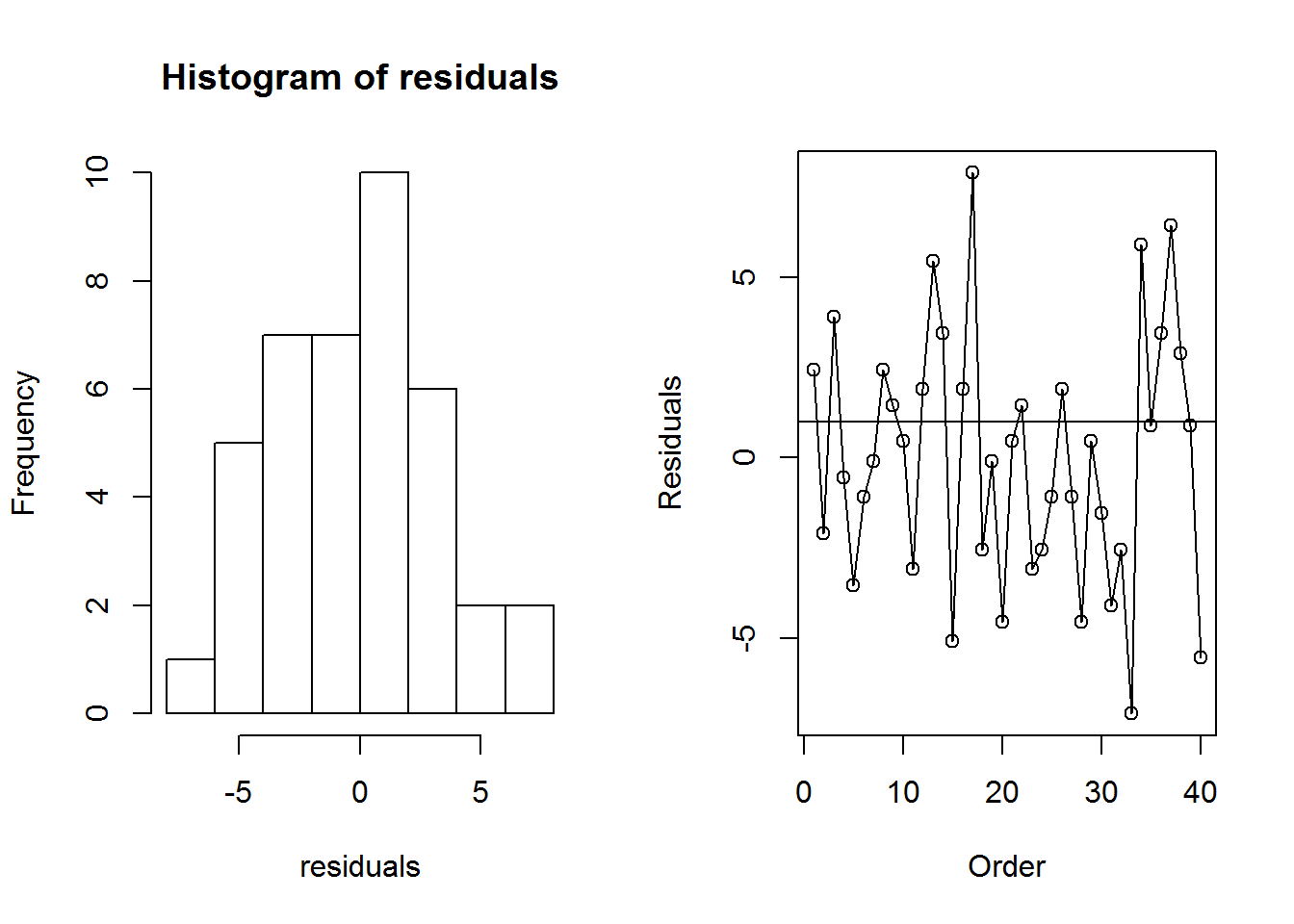#Create scatterplot of Time versus Type of game with a superimposed regression line:
plot(D1,games1$Time) abline(lm(Time~D1,data=games1)$coef)

####################
#ANOVA################
#Compute y.. , 1. y , and 2. y , respectively:
mean(games1$Time) ##  36.825 mean(Standard) ##  35.55 mean(Color) ##  38.1 #Estimate the effect size for the color distracter game: mean(Color)-mean(games1$Time)
##  1.275
#Estimate the effect size for the standard game:
mean(Standard)-mean(games1$Time) ##  -1.275 #Construct the main effects plot of game type: par(mfrow=c(1,1))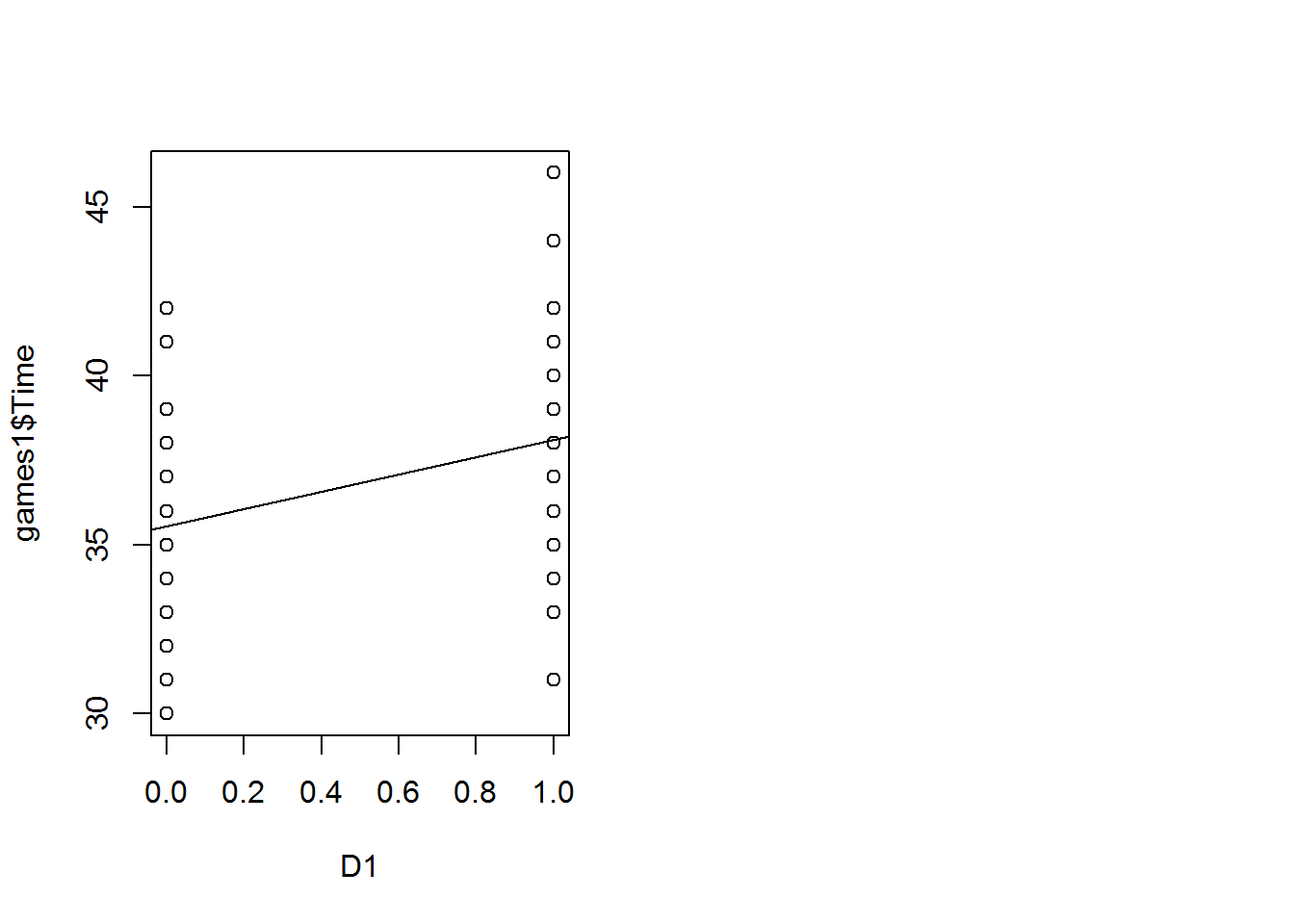plot(c(0,1),c(mean(Color),mean(Standard)),xlim=c(-.1,1.1),ylim=c(35.2,38.5), ylab="Mean Time",pch=16,xlab="Type",main="Main Effects Plot") lines(c(0,1),c(mean(Color),mean(Standard))) text(c(0,1),c(35.2,35.2),c("Color","Standard"))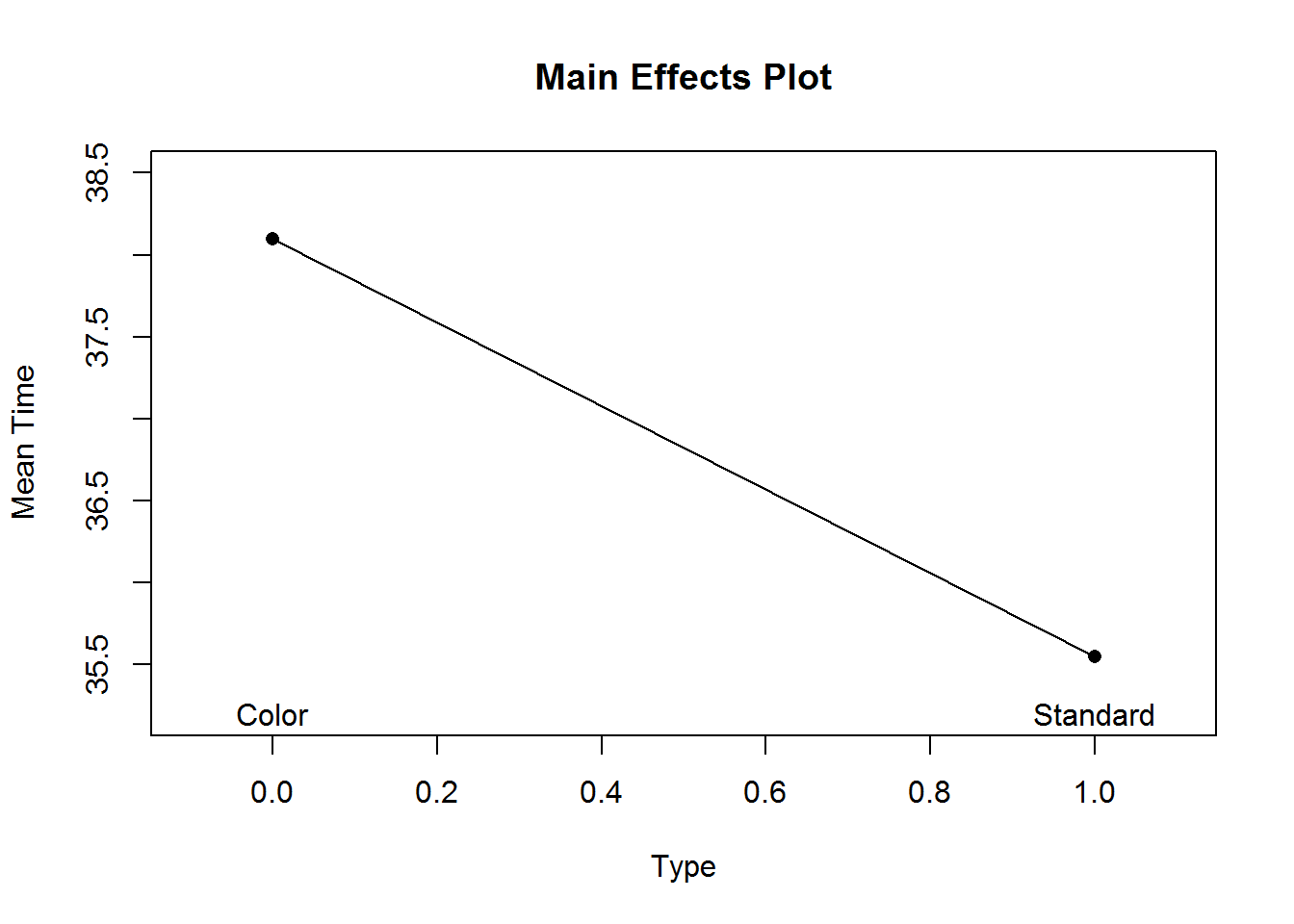#Obtain the results (F-statistic and p-value) of an analysis of variance: summary(aov(Time~Type,data=games1)) ## Df Sum Sq Mean Sq F value Pr(>F) ## Type 1 65.0 65.02 5.227 0.0279 * ## Residuals 38 472.8 12.44 ## --- ## Signif. codes: 0 '***' 0.001 '**' 0.01 '*' 0.05 '.' 0.1 ' ' 1 sqrt(5.227) ##  2.286263 # Create a vector of residuals from the analysis of variance model: residuals1 <- aov(Time~Type,data=games1)$res

#Construct a histogram of the residuals, a plot of the residuals versus type of game, and a plot of the residuals
#versus order (all in one graphics window):
par(mfrow=c(1,3))
hist(residuals1,xlab="Residuals")
#plot(games1$Type,residuals,ylab="Residuals") plot(games1$studentID,residuals,xlab="Order",ylab="Residuals")
lines(games1\$studentID,residuals1)
abline(h = 0)